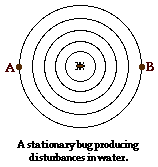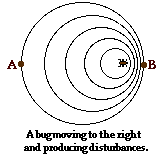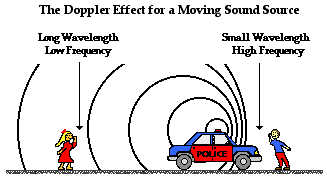Vibrations and Waves - Lesson 3 - Behavior of Waves

# The Doppler Effect

Suppose that there is a happy bug in the center of a circular water puddle. The bug is periodically shaking itslegs in order to produce disturbances that travel through the water. If these disturbances originate at a point, then they would travel outward from that point in all directions. Since each disturbance is traveling in the same medium, they would all travel in every direction at the same speed. The pattern produced by the bug's shaking would be a series of concentric circles as shown in the diagram at the right. These circles would reach the edges of the water puddle at the same frequency. An observer at point A (the left edge of the puddle) would observe the disturbances to strike the puddle's edge at the same frequency that would be observed by an observer at point B (at the right edge of the puddle). In fact, the frequency at which disturbances reach the edge of the puddle would be the same as the frequency at which the bug produces the disturbances. If the bug produces disturbances at a frequency of 2 per second, then each observer would observe them approaching at a frequency of 2 per second.

Now suppose that our bug is moving to the right across the puddle of water and producing disturbances atthe same frequency of 2 disturbances per second. Since the bug is moving towards the right, each consecutive disturbance originates from a position that is closer to observer B and farther from observer A. Subsequently, each consecutive disturbance has a shorter distance to travel before reaching observer B and thus takes less time to reach observer B. Thus, observer B observes that the frequency of arrival of the disturbances is higher than the frequency at which disturbances are produced. On the other hand, each consecutive disturbance has a further distance to travel before reaching observer A. For this reason, observer A observes a frequency of arrival that is less than the frequency at which the disturbances are produced. The net effect of the motion of the bug (the source of waves) is that the observer towards whom the bug is moving observes a frequency that is higher than 2 disturbances/second; and the observer away from whom the bug is moving observes a frequency that is less than 2 disturbances/second. This effect is known as the Doppler effect.

### What is the Doppler Effect?

The Doppler effect is observed whenever the source of waves is moving with respect to an observer. The Doppler effect can be described as the effect produced by a moving source of waves in which there is an apparent upward shift in frequency for observers towards whom the source is approaching and an apparent downward shift in frequency for observers from whom the source is receding. It is important to note that the effect does not result because of an actual change in the frequency of the source. Using the example above, the bug is still producing disturbances at a rate of 2 disturbances per second; it just appears to the observer whom the bug is approaching that the disturbances are being produced at a frequency greater than 2 disturbances/second. The effect is only observed because the distance between observer B and the bug is decreasing and the distance between observer A and the bug is increasing.

The Doppler effect can be observed for any type of wave - water wave, sound wave, light wave, etc. We are most familiar with the Doppler effect because of our experiences with sound waves. Perhaps you recall an instance in which a police car or emergency vehicle was traveling towards you on the highway. As the car approached with its siren blasting, the pitch of the siren sound (a measure of the siren's frequency) was high; and then suddenly after the car passed by, the pitch of the siren sound was low. That was the Doppler effect - an apparent shift in frequency for a sound wave produced by a moving source.### The Doppler Effect in Astronomy

The Doppler effect is of intense interest to astronomers who use the information about the shift in frequency of electromagnetic waves produced by moving stars in our galaxy and beyond in order to derive information about those stars and galaxies. The belief that the universe is expanding is based in part upon observations of electromagnetic waves emitted by stars in distant galaxies. Furthermore, specific information about stars within galaxies can be determined by application of the Doppler effect. Galaxies are clusters of stars that typically rotate about some center of mass point. Electromagnetic radiation emitted by such stars in a distant galaxy would appear to be shifted downward in frequency (a red shift) if the star is rotating in its cluster in a direction that is away from the Earth. On the other hand, there is an upward shift in frequency (a blue shift) of such observed radiation if the star is rotating in a direction that is towards the Earth.

Jump To Next Lesson:

Follow Us